Himanshi Singh — March 2, 2021

## Introduction

Regular Expressions is very popular among programmers and can be applied in many programming languages like Java, JS, php, C++, etc. Regular Expressions are useful for numerous practical day-to-day tasks that a data scientist encounters. It is one of the key concepts of Natural Language Processing that every NLP expert should be proficient in.

Regular Expressions are used in various tasks such as data pre-processing, rule-based information mining systems, pattern matching, text feature engineering, web scraping, data extraction, etc.

Note: If you are more interested in learning concepts in an Audio-Visual format, We have this entire article explained in the video below. If not, you may continue reading.

Let’s understand what regular expressions are and how we can leverage them for text feature engineering specifically in this article.

## What are Regular Expressions?

Regular expressions or RegEx is a sequence of characters mainly used to find or replace patterns embedded in the text. Let’s consider this example: Suppose we have a list of friends-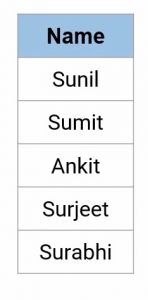And if we want to select only those names on this list which match the certain pattern such as something the like this-The names having the first two letters- S and U, followed by only three positions that can be taken up by any letter. What do you think, which names fit this criterion? Let’s go one by one, the name Sunil and Sumit fit this criterion as they have S and U in the beginning and three more letters after that. While rest of the three names are not following the given criteria as Ankit is starting with the alphabet A whereas Surjeet and Surabhi have more than three characters post S and U.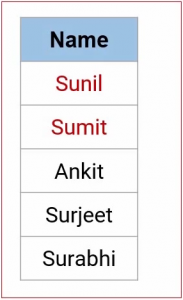What we have done here is that we have a pattern(or criteria) and a list of names and we’re trying to find the name that matches the given pattern. That’s exactly how regular expressions work.

In RegEx, we’ve different types of patterns to recognize different strings of characters. Let’s understand these terms in a bit more detail but first understand the concept of Raw Strings.

## The concept of Raw String in Regular Expression

Now let’s start with the concept of Raw String. Python raw string treats backslash(\) as a literal character. Let’s look at some examples to understand. We have a couple of backslashes here. But python treats \n as “move to a new line”.

```# normal string vs raw string

path = "C:\desktop\nathan" #string
print("string:",path)```

Let’s print this out and you’ll see what happens-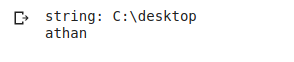As you can see, \n has moved the text after it to a new line. Here “nathon” has become “athon” and \n disappeared from the path. This is not what we want. Here we use “r” expression to create a raw string-

```path= r"C:\desktop\nathan" #raw string

print("raw string:",path)```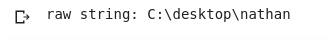As you can see we have the entire path printed out here by simply using “r” in front of the path.

It is always recommended to use raw string while dealing with Regular expressions.

## Python Built-in Module for Regular Expressions

Python has a built-in module to work with regular expressions called “re”. Some common methods from this module are-

• re.match()
• re.search()
• re.findall()

Let us look at each method with the help of an example-

#### 1. re.match(pattern, string)

The re.match function returns a match object on success and none on failure.

```import re

#match a word at the beginning of a string

result = re.match('Analytics',r'Analytics Vidhya is the largest data science community of India')
print(result)

```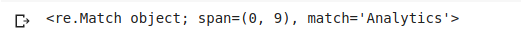Here Pattern = ‘Analytics’ and String = ‘Analytics Vidhya is the largest data science community of India’. Since the pattern is present at the beginning of the string we got the matching Object as an output. And since the output of the re.match is an object, we will use the group() function of the match object to get the matched expression.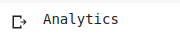As you can see, we got our required output using the group() function. Now let us have a look at the other case as well-

```result_2 = re.match('largest',r'Analytics Vidhya is the largest data science community of India')
print(result_2)```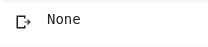Here as you can notice, our pattern(largest) is not present at the beginning of the string, hence we got None as our output.

#### 2. re.search(pattern, string)

Matches the first occurrence of a pattern in the entire string(and not just at the beginning).

```# search for the pattern "founded" in a given string

result = re.search('founded',r'Andrew NG founded Coursera. He also founded deeplearning.ai')
print(result.group())```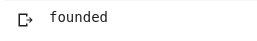Since our pattern(founded) is present in the string, re.search() has matched the single occurrence of the pattern.

#### 3. re.findall(pattern, string)

It will return all the occurrences of the pattern from the string. I would recommend you to use re.findall() always, it can work like both re.search() and re.match().

```result = re.findall('founded',r'Andrew NG founded Coursera. He also founded deeplearning.ai')
print(result)```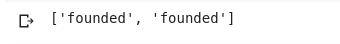Since we’ve ‘founded’ twice here in the string, re.findall() has printed it out twice in the output.

## Special Sequences in Regular Expressions

Now we’re going to look at some special sequences that come up with Regular expressions. These are used to extract a different kind of information from a given text. Let’s take a look at them-

#### 1. \b

\b returns a match where the specified pattern is at the beginning or at the end of a word.

```str = r'Analytics Vidhya is the largest Analytics community of India'

#Check if there is any word that ends with "est"

x = re.findall(r"est\b", str)
print(x)```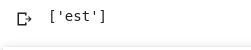As you can see it returned the last three characters of the word “largest”.

#### 2. \d

\d returns a match where the string contains digits (numbers from 0-9).

```str = "2 million monthly visits in Jan'19."

#Check if the string contains any digits (numbers from 0-9):

x = re.findall("\d", str)
print(x)

if (x):
print("Yes, there is at least one match!")
else:
print("No match")```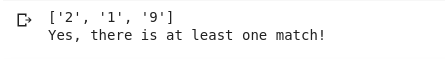This function has generated all the digits from the string i.e 2, 1, and 9 separately. But is this what we want? I mean, 1and 9 were together in the string but in our output we got 1 and 9 separated. Let’s see how we can get our desired output-

```str = "2 million monthly visits in Jan'19."

# Check if the string contains any digits (numbers from 0-9):
# adding '+' after '\d' will continue to extract digits till encounters a space

x = re.findall("\d+", str)
print(x)

if (x):
print("Yes, there is at least one match!")
else:
print("No match")```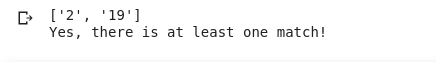We can solve this problem by using the ‘+’ sign. Notice how we used ‘\d+’ instead of ‘\d’. Adding ‘+’ after ‘\d’ will continue to extract the digits till we encounter a space. We can infer that \d+ repeats one or more occurrences of \d till the non-matching character is found whereas \d does a character-wise comparison.

#### 3. \D

\D returns a match where the string does not contain any digit. It is basically the opposite of \d.

```str = "2 million monthly visits in Jan'19."

#Check if the word character does not contain any digits (numbers from 0-9):

x = re.findall("\D", str)
print(x)

if (x):
print("Yes, there is at least one match!")
else:
print("No match")```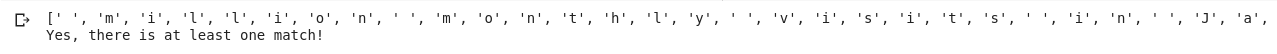We’ve got all the strings where there are no digits. But again we are getting individual characters as output and like this, they really don’t make sense. By now I believe you know how to tackle this problem now-

```#Check if the word does not contain any digits (numbers from 0-9):

x = re.findall("\D+", str)
print(x)

if (x):
print("Yes, there is at least one match!")
else:
print("No match")```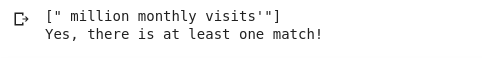Bingo! use \D+ instead of just \D to get characters that make sense.

#### 4. \w

\w helps in extraction of alphanumeric characters only (characters from a to Z, digits from 0-9, and the underscore _ character)

```str = "2 million monthly visits!"

#returns a match at every word character (characters from a to Z, digits from 0-9, and the underscore _ character)

x = re.findall("\w+",str)
print(x)

if (x):
print("Yes, there is at least one match!")
else:
print("No match")```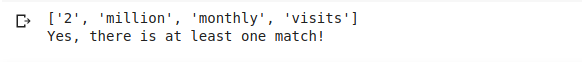We got all the alphanumeric characters.

#### 5. \W

\W returns match at every non-alphanumeric character. Basically opposite of \w.

```str = "2 million monthly visits9!"

#returns a match at every NON word character (characters NOT between a and Z. Like "!", "?" white-space etc.):

x = re.findall("\W", str)
print(x)

if (x):
print("Yes, there is at least one match!")
else:
print("No match")```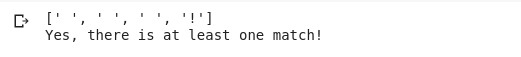We got every non-alphanumeric character including white spaces.

## Metacharacters in Regular Expression

Metacharacters are characters with a special meaning.

#### 1- (.) matches any character (except newline character)

```str = "rohan and rohit recently published a research paper!"

#Search for a string that starts with "ro", followed by any number of characters

x = re.findall("ro.", str)           #searches one character after ro
x2 = re.findall("ro...", str)        #searches three characters after ro

print(x)
print(x2)```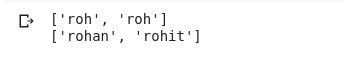We got “roh” and “roh” as our first output since we used only one dot after “ro”. Similarly, “rohan” and “rohit” as our second output since we used three dots after “ro” in the second statement.

#### 2–(^) starts with

It checks whether the string starts with the given pattern or not.

```str = "Data Science"

#Check if the string starts with 'Data':

x = re.findall("^Data", str)

if (x):
print("Yes, the string starts with 'Data'")
else:
print("No match")```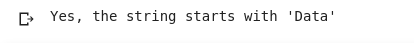This caret(^) symbol checked whether the string started with “Data” or not. And since our string is starting with the word Data, we got this output. Let’s check the other case as well-
```# try with a different string

str2 = "Big Data"

#Check if the string starts with 'Data':

x2 = re.findall("^Data", str2)

if (x2):
print("Yes, the string starts with 'data'")
else:
print("No match")```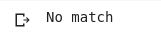Here in this case you can see that the new string is not starting with the word “Data”, hence we got No match.

#### 3- (\$) ends with

It checks whether the string ends with the given pattern or not.

```str = "Data Science"

#Check if the string ends with 'Science':

x = re.findall("Science\$", str)

if (x):
print("Yes, the string ends with 'Science'")
else:
print("No match")```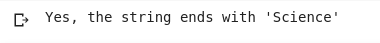The dollar(\$) sign checks whether the string ends with the given pattern or not. Here, our pattern is Science and since the string ends with Science we got this output.

#### 4- (*) matches for zero or more occurrences of the pattern to the left of it

```str = "easy easssy eay ey"

#Check if the string contains "ea" followed by 0 or more "s" characters and ending with y

x = re.findall("eas*y", str)
print(x)

if (x):
print("Yes, there is at least one match!")
else:
print("No match")```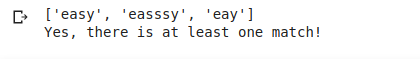The above code block basically checks if the string contains the pattern”eas*y” this means “ea” followed by one or more occurrences of “s” and ending with “y”. We got these three strings as output -” easy”, “easssy”, and “eay” because they match the given pattern. But the string “ey” does not contain the pattern we’re looking for.

#### 5- (+) matches one or more occurrences of the pattern to the left of it

```#Check if the string contains "ea" followed by 1 or more "s" characters and ends with y

x = re.findall("eas+y", str)
print(x)

if (x):
print("Yes, there is at least one match!")
else:
print("No match")```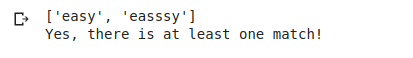One major difference between * and + is that + checks for one or more occurrences of the pattern to the left of it. Like in this above example we got “easy” and “easssy” as output but not “eay” and “ey” because “eay” does not contain any instance of the character “s” and “ey” has already been discarded earlier.

#### 6- (?) matches zero or one occurrence of the pattern left to it.

```x = re.findall("eas?y",str)

print(x)

if (x):
print("Yes, there is at least one match!")
else:
print("No match")```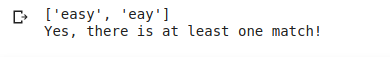The question mark(?) looks for zero or one occurrence of the pattern to the left of it. That is why we got “easy” and “eay” as our output since only these two strings contains one and zero occurrence of the character “s” respectively, along with the pattern starting with “ea” and ending with “y”.

#### 7- (|) either or

```str = "Analytics Vidhya is the largest data science community of India"

#Check if the string contains either "data" or "India":

x = re.findall("data|India", str)
print(x)

if (x):
print("Yes, there is at least one match!")
else:
print("No match")```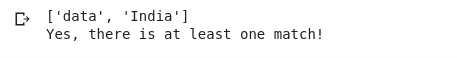The pipe(|) operator checks whether any of the two patterns, to its left and right, is present in the String or not. Here in the above example, we’re checking the String either contains data or India. Since both of them are present in the String, we got both as the output.

Let’s look at another example:

```# try with a different string

str = "Analytics Vidhya is one of the largest data science communities"

#Check if the string contains either "data" or "India":

x = re.findall("data|India", str)
print(x)

if (x):
print("Yes, there is at least one match!")

else:
print("No match")```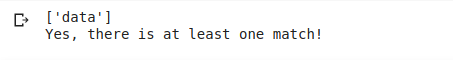Here the pattern is the same but the String contains only “data” and hence we got only [‘data’] as the output.

## End Notes

In this article, I covered basic concepts of RegEx in detail including the concept of raw string, the re module and some of its functions, special sequences, and metacharacters in Regular Expression.

Regular Expressions are really important if you deal with unstructured text data on daily basis.

I recommend you check out our free python course, Introduction to Python to learn Regular Expressions and more interesting topics.

Reach out to us in the comments below in case you have any doubts.